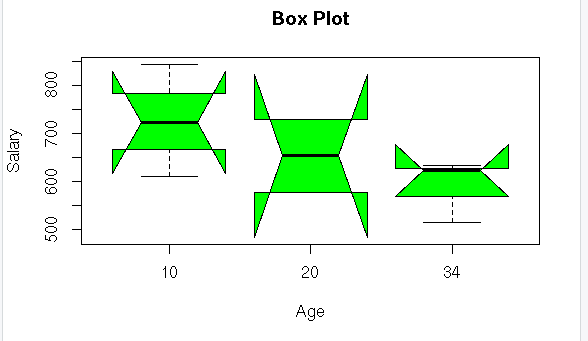• #5, First Floor, 4th Street Dr. Subbarayan Nagar Kodambakkam, Chennai-600 024 Landmark : Samiyar Madam
• pro@slogix.in
• +91- 81240 01111

### How to create boxplot in R?

###### Description

To create a boxplot for the given data using R programming.

#### Boxplot:

Measure how well data is distributed in a data set.

Divides the data set into three quartiles.

Represents the minimum, maximum, median, first quartile and third quartile in the data set.

Useful in comparing the distribution of data across data sets by drawing boxplots for each of them.

R Function : boxplot()

###### Sapmle Code

#Data Visualization in Box plot

print(getwd())
setwd(“/home/soft13″)
print(getwd())